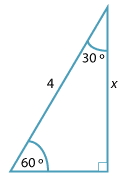#### Question 2Detailed description

 a b c $$\dfrac{x}{4}$$ is? sin 30° sin 60° tan 60° The value of $$\dfrac{x}{4}$$ is? $$\dfrac{1}{2}$$ $$\sqrt{3}$$ $$\dfrac{\sqrt{3}}{2}$$ The exact value of $$x$$ is? $$2\sqrt{3}$$ 2 $$4\sqrt{3}$$